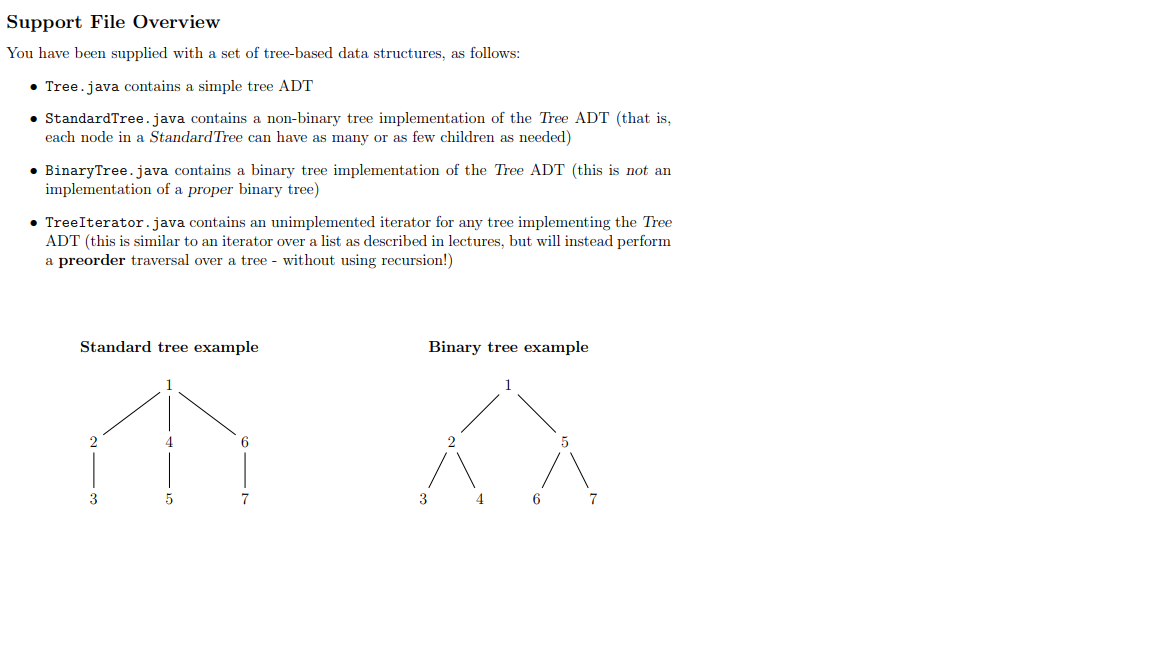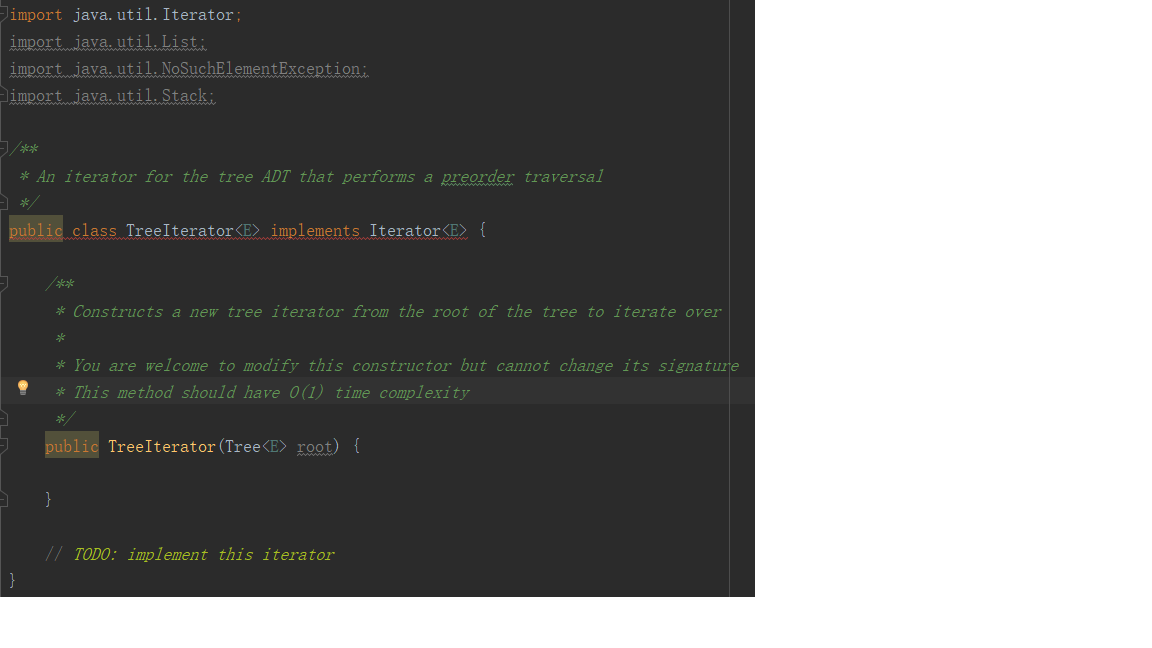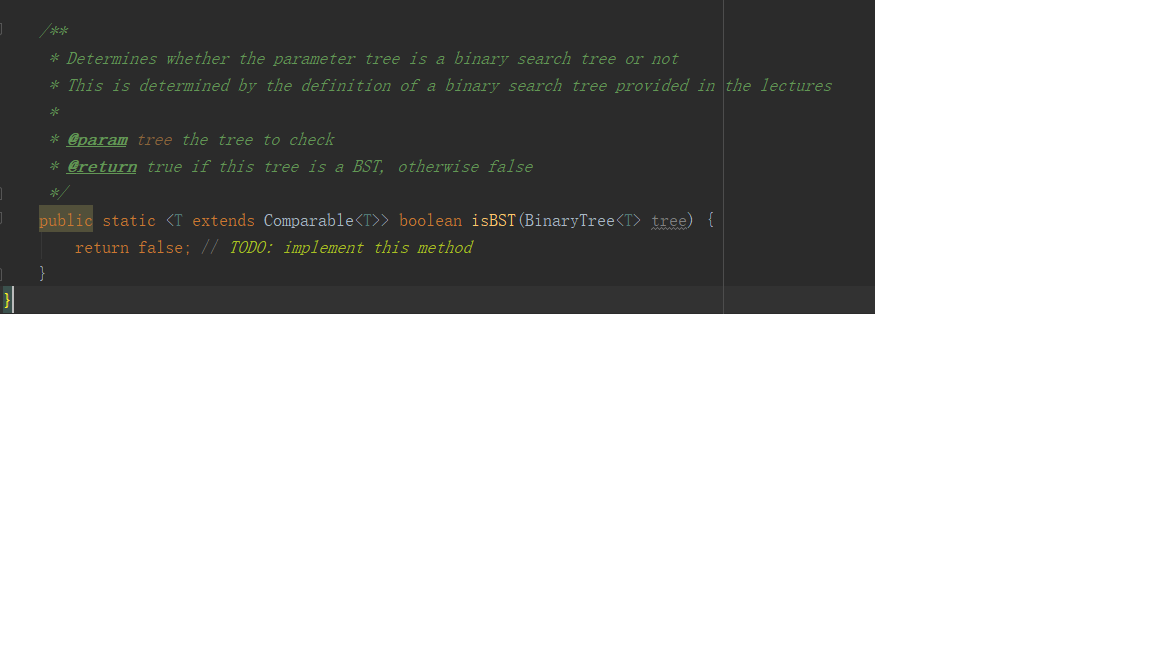# question 1 implement the treeiterator class so that a preorder traversal of any tree 5153911

Question:

1. Implement the TreeIterator class, so that a preorder traversal of any tree implementing the Tree ADT can be performed using this iterator. At a minimum, you will need to implement the next and hasNext methods as per their specifications in the Iterator interface.

2. Implement the isBST method as per its Javadoc specification. The method should return whether a given binary tree is a binary search tree or not. You may write one or more private helper methods as a part of your solution.

3. In the Javadoc comments for each of the isBST, hasNext, and next methods, state (in big-O notation) and briefly explain the worst-case time complexity of a single call to each of these methods. If the amortised time complexity of any of these methods differ, also provide that and explain why it differs.

4. Trees have a variety of practical applications. For this question, you will be required to model a practical scenario that makes use of trees, as follows: • Write another class (in a separate Java file) that models your chosen practical scenario and implements/extends/utilises one of the given tree ADTs or CDTs. Your implementation should introduce or override at least two methods. Each of these methods should have non-trivial functionality and be documented appropriately. • In the Javadoc comment for this class, explain why a tree is a useful data structure to model your chosen scenario. • In the file MyTreeTest.java, write at least two realistic JUnit tests that demonstrate the usage of this data structure and its methods.

Support Files :Q1. /** * An iterator for the tree ADT that performs a preorder traversal */ public class TreeIterator implements Iterator { /** * Constructs a new tree iterator from the root of the tree to iterate over * * You are welcome to modify this constructor but cannot change its signature * This method should have O(1) time complexity */ public TreeIterator(Tree root) { } // TODO: implement this iterator }Q2.

/** * A binary tree, where each node contains at most two children * Each root node contains a value and references to its left and right children (if they exist) * * @param the type of the tree&#39;s elements */ public class BinaryTree implements Tree { private E root; // the element sitting at this tree&#39;s root node private BinaryTree left; // the left child (subtree) private BinaryTree right; // the right child (subtree) /** * Constructs a new binary tree with a single node * * @param root the element to store at this tree&#39;s root */ public BinaryTree(E root) { this.root = root; this.left = null; this.right = null; }

………

/** * Determines whether the parameter tree is a binary search tree or not * This is determined by the definition of a binary search tree provided in the lectures * * @param tree the tree to check * @return true if this tree is a BST, otherwise false */ public static > boolean isBST(BinaryTree tree) { return false; // TODO: implement this method }Support File Overview You have been supplied with a set of tree-based data structures, as follows: • Tree.java contains a simple tree ADT • StandardTree.java contains a non-binary tree implementation of the Tree ADT (that is, each node in a Standard Tree can have as many or as few children as needed) • Binary Tree.java contains a binary tree implementation of the Tree ADT (this is not an implementation of a proper binary tree) TreeIterator.java contains an unimplemented iterator for any tree implementing the Tree ADT (this is similar to an iterator over a list as described in lectures, but will instead perform a preorder traversal over a tree – without using recursion!) Standard tree example Binary tree example 4 6 import java.util. Iterator; importmjava.util.Listä import java. util. NoSuchElementException: import java. util. Stacki * An iterator for the tree ADT that performs a preorder traversal public class TreeIterator {/ * Constructs a new tree iterator from the root of the tree to iterate over * You are welcome to modify this constructor but cannot change its signature * This method should have 0(1) time complexity public TreeIterator (Tree root) { // TODO: implement this iterator * Determines whether the parameter tree is a binary search tree or not This is determined by the definition of a binary search tree provided in the lectures @param tree the tree to check @return true if this tree is a BST, otherwise false public static > boolean isBST (BinaryTree tree) { return false; // TODO: implement this method